Name:    Chapter 6 Pre Test

Multiple Choice
Identify the choice that best completes the statement or answers the question.

1.

Given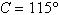,, and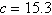, use the Law of Sines to solve the triangle (if possible) for the value of b. If two solutions exist, find both. Round answer to two decimal places.
 a.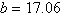b.c.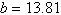d.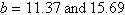e. not possible

2.

Determine a value for b such that a triangle with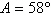and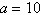has no solution. Round your answer to the nearest hundredth.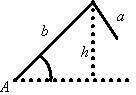a. 11.69 b. 2.36 c. 2.95 d. 5.9 e. 31.83

3.

Determine the area of a triangle having the following measurements. Round your answer to two decimal places.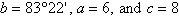a. 23.84 sq. units b. 28.61 sq. units c. 21.46 sq. units d. 19.07 sq. units e. 26.22 sq. units

4.

Given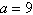,, and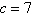, use the Law of Cosines to solve the triangle for the value of B. Round answer to two decimal places.
 a.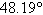b.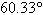c.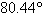d.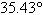e.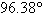5.

In the figure below,,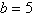, and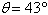. Use this information to solve the parallelogram for c. The diagonals of the parallelogram are represented by c and d. Round answer to two decimal places.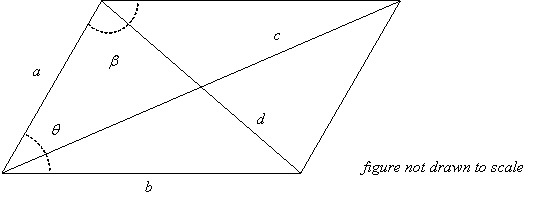a. 5.52 b. 12.15 c. 10.49 d. 9.25 e.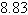6.

In the figure below,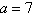,, and. Use this information to solve the parallelogram for b. The diagonals of the parallelogram are represented by c and d. Round answer to two decimal places.a. 12.77 b. 6.03 c. 11.08 d. 9.82 e. 9.4

7.

Find the component form of vector v with initial point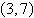and terminal point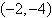.
 a.b.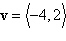c.d.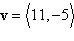e.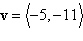8.

Find a unit vector in the direction of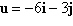.
 a.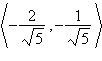b.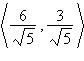c.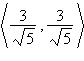d.e.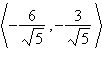9.

Find the magnitude and direction angle of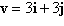. Round direction angle to nearest degree.
 a.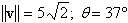b.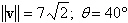c.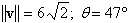d.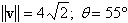e.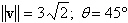10.

Find the component form of v if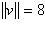and the angle it makes with the x-axis is 150°.
 a.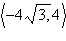b.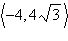c.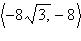d.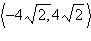e.11.

Three forces with magnitudes of 95 pounds, 75 pounds, and 146 pounds act on an object at angles 100°, 200°, and 300°, respectively, with the positive x-axis. Find the magnitude and direction of the resultant force. Round answers to two decimal places.
 a. 41.84 pounds; 256.57° b. 41.84 pounds; 76.57° c. 60.18 pounds; 256.57° d. 60.18 pounds; 76.57° e. 81.89 pounds; 288.09°

12.

Given vectors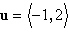and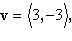determine the quantity indicated below.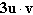a. –27 b. –9 c. –24 d. 3 e. –15

13.

A force of 45 pounds is exerted along a rope attached to a crate at an angle of 30° above the horizontal. The crate is moved 23 feet. How much work has been accomplished? Round answer to one decimal place.
 a. 1,035.0 foot-pounds b. 896.3 foot-pounds c. 1,195.1 foot-pounds d. 965.7 foot-pounds e. 1,080.4 foot-pounds

14.

Find the trigonometric form of the complex number represented graphically.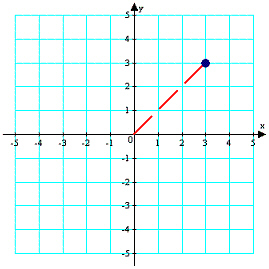a.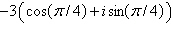b.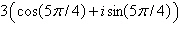c.d.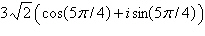e.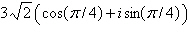15.

Find the trigonometric form of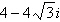.
 a.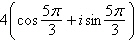b.c.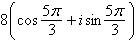d.e.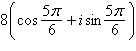16.

Perform the operation shown below and leave the result in trigonometric form.a.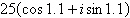b.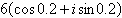c.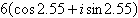d.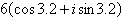e.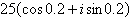17.

Find the product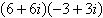using trigonometric forms. Leave the result in trigonometric form.
 a.b.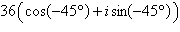c.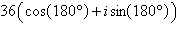d.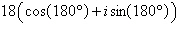e.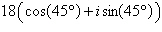18.

Use DeMoivre's Theorem to find the indicated power of the complex number. Write the result in standard form.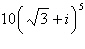a.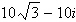b.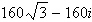c.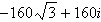d.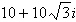e.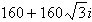19.

Use DeMoivre's Theorem to find the indicated power of the following complex number.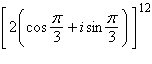a.b. –4,096 c. 2048 d. 4,096 e.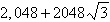20.

Find the fourth roots of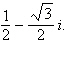Write the roots in trigonometric form.
 a.b.c.d.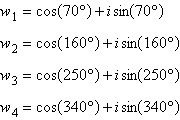e.